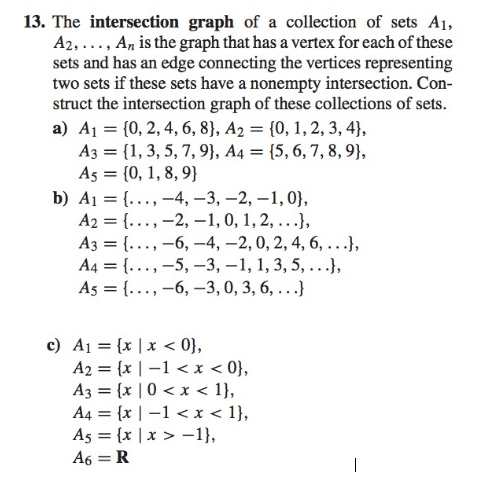# Question The intersection graph of a collection of sets A1, A2, ....... An is the graph that has a vertex for each of these sets and has an edge connecting the vertices representing two sets if these sets have a nonempty intersection. Construct the intersection graph of these collections of sets. A1 = (0, 2, 4, 6, 8), A2 = (0, 1, 2, 3, 4), A3 = (1, 3, 5, 7, 9), A4 = The intersection graph of a collection of sets A1, A2, ....... An is the graph that has a vertex for each of these sets and has an edge connecting the vertices representing two sets if these sets have a nonempty intersection. Construct the intersection graph of these collections of sets. A1 = (0, 2, 4, 6, 8), A2 = (0, 1, 2, 3, 4), A3 = (1, 3, 5, 7, 9), A4 = (5, 6, 7, 8, 9), A5 = (0, 1, 8, 9) A1 = (......, -4, -3, -2, 1, 0), A2 = (....., -2, 1, 0, 1, 2, ...) A3 = (....., -6, -4, 2, 0, 2, 4, 6 ....) A4 = (......, -5, -3, 1, 1, 3, 5, ....., ) A5 = (.......- 6, -3, 0, 3, 6, ....) A1 = (x l x < 0), A2 = (x|-1 < x <0) A3 = (x| 0 < x < 1) A4 = x| -1 < x < 1) A5 = (x| x > -1) A6 = R1JWVFL The Asker · Other MathematicsTranscribed Image Text: The intersection graph of a collection of sets A1, A2, ....... An is the graph that has a vertex for each of these sets and has an edge connecting the vertices representing two sets if these sets have a nonempty intersection. Construct the intersection graph of these collections of sets. A1 = (0, 2, 4, 6, 8), A2 = (0, 1, 2, 3, 4), A3 = (1, 3, 5, 7, 9), A4 = (5, 6, 7, 8, 9), A5 = (0, 1, 8, 9) A1 = (......, -4, -3, -2, 1, 0), A2 = (....., -2, 1, 0, 1, 2, ...) A3 = (....., -6, -4, 2, 0, 2, 4, 6 ....) A4 = (......, -5, -3, 1, 1, 3, 5, ....., ) A5 = (.......- 6, -3, 0, 3, 6, ....) A1 = (x l x < 0), A2 = (x|-1 < x <0) A3 = (x| 0 < x < 1) A4 = x| -1 < x < 1) A5 = (x| x > -1) A6 = R
More
Transcribed Image Text: The intersection graph of a collection of sets A1, A2, ....... An is the graph that has a vertex for each of these sets and has an edge connecting the vertices representing two sets if these sets have a nonempty intersection. Construct the intersection graph of these collections of sets. A1 = (0, 2, 4, 6, 8), A2 = (0, 1, 2, 3, 4), A3 = (1, 3, 5, 7, 9), A4 = (5, 6, 7, 8, 9), A5 = (0, 1, 8, 9) A1 = (......, -4, -3, -2, 1, 0), A2 = (....., -2, 1, 0, 1, 2, ...) A3 = (....., -6, -4, 2, 0, 2, 4, 6 ....) A4 = (......, -5, -3, 1, 1, 3, 5, ....., ) A5 = (.......- 6, -3, 0, 3, 6, ....) A1 = (x l x < 0), A2 = (x|-1 < x <0) A3 = (x| 0 < x < 1) A4 = x| -1 < x < 1) A5 = (x| x > -1) A6 = R